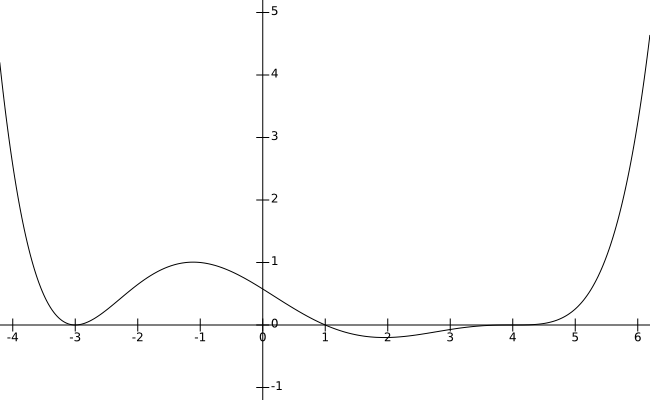# Section 3.2 - Polynomials: Graphs and Properties

Section Objectives

1. Find the zeros of a polynomial and determine their multiplicities.
2. Determine the end behavior of a polynomial function.
3. Use intercepts and end behavior to graph a polynomial function.

### Polynomials

An $n$-th-degree polynomial in the variable $x$ is a function of the form

$f(x)=a_nx^n+a_{n-1}x^{n-1}+\cdots+a_1x+a_0$,

where the coefficients $a_n$, $a_{n-1}$, $\dots$, $a_0$ are real or complex numbers with $a_n \ne 0$.

The zeros of a function $f$ are those $x$-values for which $f(x)=0$. The real zeros of $f$ correspond to the $x$-intercepts of the graph of $f$: if the number $k$ is a zero of $f$, then $(k,0)$ is an $x$-intercept of the graph.

To find the zeros of a polynomial function, we must solve a polynomial equation of the form

$a_nx^n+a_{n-1}x^{n-1}+\cdots+a_1x+a_0=0.$

General polynomial equations are often solved by first making one side of the equation equal to zero. After doing so, the next steps may vary depending on the nature of the polynomial. For now, we will focus on solving by factoring.

### Factored polynomials and zeros

When a polynomial is completely factored, the number of times a specific linear factor occurs in the factorization is called the multiplicity of the corresponding zero.

#### Examples

• $g(x)=4(x-1)^3(x+5)(x+8)^2$ has zeros $x=1$, $x=-5$, and $x=-8$, with multiplicities 3, 1, and 2, respectively.

• Let $f(x)=x^7(x-1)^5(x+2)$. Find the zeros of $f$ and their multiplicities.

• Find the zeros (and their multiplicities) of $f(x)=x^2+8x+16$.

### End behavior, zeros, and polynomial graphs

The general shape of the graph of a polynomial can be easily determined from the polynomial's factored form.

• The graph crosses the $x$-axis at every zero of multiplicity 1.

• The graph flattens and crosses the $x$-axis at every zero of odd multiplicity.

• The graph flattens, touches, and bounces off the $x$-axis at every zero of even multiplicity.

• The end behavior (the behavior as $x \to \pm \infty$) of the graph of is identical to that of $y=a_nx^n$, where $n$ is the degree and $a_n$ is the leading coefficient:

• $n$ even and $a_n$ positive $\longrightarrow$ up left and up right
• $n$ even and $a_n$ negative $\longrightarrow$ down left and down right
• $n$ odd and $a_n$ positive $\longrightarrow$ down left and up right
• $n$ odd and $a_n$ negative $\longrightarrow$ up left and down right

#### Example

• Here is the graph of $f(x)=0.001(x-4)^3(x-1)(x+3)^2$.• Discuss the zeros and the features of the graph of $g(x)=x^2(x-3)^5$.

• Imagine you were given the graph of a polynomial function, but you were not given the polynomial itself. Could you make good predictions about the zeros and their multiplicities?